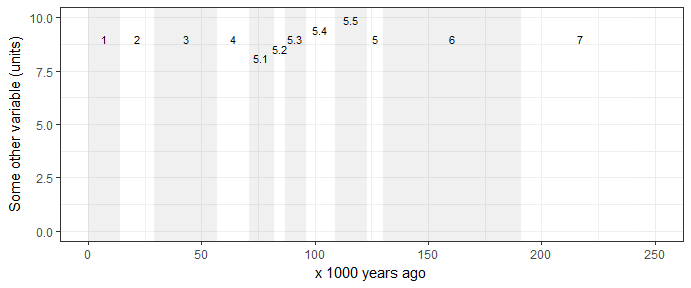# Introduction

## Overview

This package includes commonly used datasets in palaeoecology and archaeology:

• Global sea level curve
• Global oxygen isotope curve
• Boundary ages for Marine Isotope Stages

There are many possible sources for these kinds of data, this package includes data from:

Dataset Source
lisiecki2005 Lisiecki, L.E. and M.E. Raymo. 2005. A Pliocene-Pleistocene stack of 57 globally distributed benthic D18O records. Paleoceanography, Vol. 20, PA1003, https://doi.org/10.1029/2004PA001071
spratt2016 Spratt, Rachel M. and Lorraine E. Lisiecki 2016. A Late Pleistocene sea level stack. Climate of the Past. Vol. 12, 1079-1092, https://doi.org/10.5194/cp-12-1-2016

You must cite those sources in the table above if you use the data in this package.

Here’s the structure of the main data sets:

library(gsloid)
str(lisiecki2005)
## 'data.frame':    2115 obs. of  3 variables:
##  $Time : num 0 1 2 3 4 5 6 7 8 9 ... ##$ d18O : num  3.23 3.23 3.18 3.29 3.3 3.26 3.33 3.37 3.42 3.38 ...
##  $Error: num 0.03 0.04 0.03 0.03 0.03 0.03 0.04 0.04 0.03 0.04 ... str(spratt2016) ## 'data.frame': 799 obs. of 9 variables: ##$ age_calkaBP            : num  0 1 2 3 4 5 6 7 8 9 ...
##  $SeaLev_shortPC1 : num 8.49 7.63 4.01 4.35 3.13 ... ##$ SeaLev_shortPC1_err_sig: num  5.23 4.87 4.83 4.72 4.74 4.57 5.04 5.9 6.79 8.3 ...
##  $SeaLev_shortPC1_err_lo : num -1.72 -2.9 -4.51 -6.93 -10.43 ... ##$ SeaLev_shortPC1_err_up : num  17.93 16.39 13.59 12.08 8.41 ...
##  $SeaLev_longPC1 : num 8.96 7.72 5.96 3.54 1.88 0 -2 -5.38 -7.12 -11.6 ... ##$ SeaLev_longPC1_err_sig : num  5.72 5.13 4.69 4.42 4.39 ...
##  $SeaLev_longPC1_err_lo : num -1.21 -2.77 -5.01 -7.28 -10.54 ... ##$ SeaLev_longPC1_err_up  : num  20.38 17.1 14.21 10.9 7.63 ...
str(LR04_MISboundaries)
## 'data.frame':    232 obs. of  7 variables:
##  $MIS_Boundary : chr "1/2" "2/3" "3/4" "4/5" ... ##$ start_MIS        : chr  "1" "2" "3" "4" ...
##  $end_MIS : chr "2" "3" "4" "5" ... ##$ label_MIS        : chr  "1" "2" "3" "4" ...
##  $LR04_Age_ka_start: num 14 29 57 71 82 87 96 109 123 130 ... ##$ LR04_Age_ka_end  : num  0 14 29 57 71 82 87 96 109 123 ...
##  $LR04_Age_ka_mid : num 7 21.5 43 64 76.5 ... Detailed descriptions of the variables are avaliable in the data documentation, run ?lisiecki2005, ?spratt2016 and ?LR04_MISboundaries for more information. ## Usage Atlhough these data are suitable for many kinds of analyses, the primary reason that I made this package is so I can easily make my own plots of these data. Here’s how I typically start with plotting the oxygen isotope data. I have set limits on the x-axis so it only shows 0-250 ka because that’s what I’m interested in: library(ggplot2) ggplot(lisiecki2005, aes(Time, d18O)) + geom_line() + scale_x_continuous(limits = c(0, 250), name = "x 1000 years ago") + scale_y_reverse(name = bquote(delta^18*O)) + theme_bw()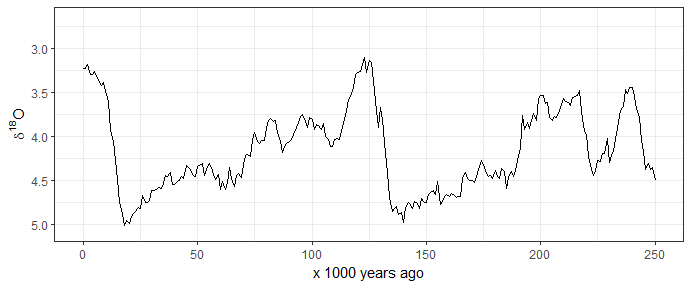And here’s how I start to plot the sea level data: ggplot(spratt2016, aes(age_calkaBP, SeaLev_shortPC1)) + geom_line() + scale_x_continuous(limits = c(0, 250), name = "x 1000 years ago") + scale_y_continuous(name = "Sea Level, meters above present day") + theme_bw()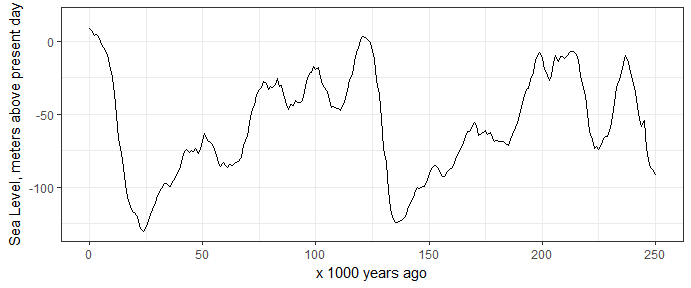Often we want to see the Marine istope stages on these plots also. This package includes the dataset LR04_MISboundaries of start and end dates for each stage, so we can draw these stages easily. I obtained these data from Lorraine Lisiecki’s web page: http://lorraine-lisiecki.com/LR04_MISboundaries.txt Some care is required to get the MIS numbers positions in easy-to-read locations. Here I use rep() and seq() to help position the numbers. # subset the MIS data for the last 250 ka years mis_last_250ka <- LR04_MISboundaries[LR04_MISboundaries$LR04_Age_ka_start <= 250, ]

# plot the oxygen istope data line on top of the MIS lines
ggplot() +
geom_vline(data = mis_last_250ka,          # add MIS lines
aes(xintercept = LR04_Age_ka_start),
colour = "blue") +
annotate("text",
label = mis_last_250ka$label_MIS, x = mis_last_250ka$LR04_Age_ka_mid,
y = c(rep(3.0, 4),
seq(3.2, 2.7, length.out = 6),
rep(3.0, 2)),
size = 3) +
geom_line(data = lisiecki2005,                # add d18O line
aes(Time,
d18O)) +
scale_x_continuous(limits = c(0, 250),
name = "x 1000 years ago") +
scale_y_reverse(name = bquote(delta^18*O)) +
theme_bw()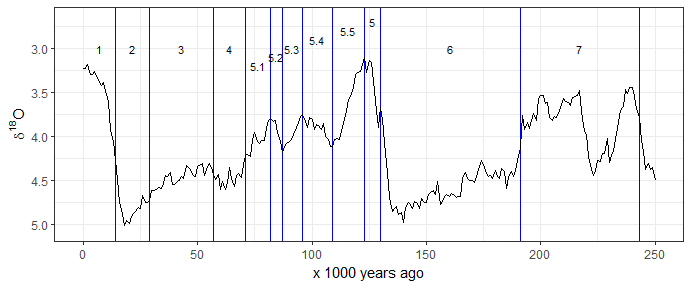Sometimes we prefer to indicate the MIS by horizontal lines. Once again we have to carefully place the line segments and labels so they are clear to read:

ggplot() +
geom_segment(data = mis_last_250ka, # add MIS lines
aes(x =    LR04_Age_ka_end,
xend = LR04_Age_ka_start,
y =    seq(3, 2.5, length.out = nrow(mis_last_250ka)),
yend = seq(3, 2.5, length.out = nrow(mis_last_250ka))),
colour = "blue",
size = 1) +
annotate("text",
label = mis_last_250ka$label_MIS, x = mis_last_250ka$LR04_Age_ka_mid,
y = c(seq(2.9, 2.7, length.out = 4),
seq(3.1, 2.8, length.out = 5),
seq(2.4, 2.3, length.out = 3)),
size = 3) +
geom_line(data = lisiecki2005,                # add d18O line
aes(Time,
d18O)) +
scale_x_continuous(limits = c(0, 250),
name = "x 1000 years ago") +
scale_y_reverse(name = bquote(delta^18*O)) +
theme_bw()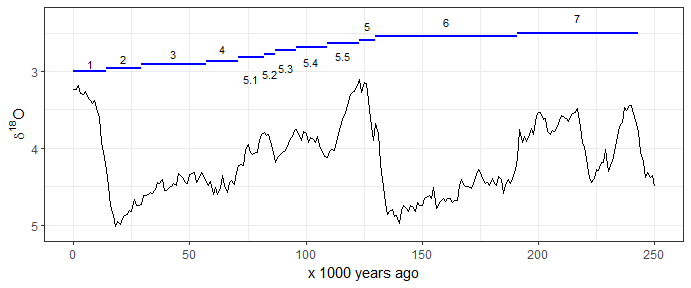And sometimes we might want shaded rectangles to indicate the MIS:

ggplot() +
annotate("rect",
xmin = mis_last_250ka$LR04_Age_ka_end, xmax = mis_last_250ka$LR04_Age_ka_start,
ymin = -Inf,
ymax = Inf,
alpha = .2,
fill = rep(c("grey70", "white"),
nrow(mis_last_250ka)/2)) +
annotate("text",
label = mis_last_250ka$label_MIS, x = mis_last_250ka$LR04_Age_ka_mid,
y = c(rep(3, 4),
seq(3.1, 2.8, length.out = 5),
rep(3, 3)),
size = 3) +
geom_line(data = lisiecki2005,                # add d18O line
aes(Time,
d18O)) +
scale_x_continuous(limits = c(0, 250),
name = "x 1000 years ago") +
scale_y_reverse(name = bquote(delta^18*O)) +
theme_bw()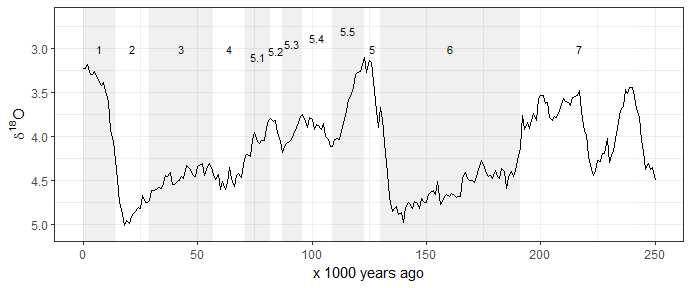Maybe we want the MIS regions by themselves so we can plot some other time series besides the ones includes here. We need to adjust the y values in the annotate() function to ensure that the MIS labels show in a readble way. Here I show an imaginary variable that might have a maximum value of about 10. So I adjust the y values in the annotate() function to position the MIS labels just below 10 on the y axis.

ggplot() +
annotate("rect",
xmin = mis_last_250ka$LR04_Age_ka_end , xmax = mis_last_250ka$LR04_Age_ka_start,
ymin = -Inf,
ymax = Inf,
alpha = .2,
fill = rep(c("grey70", "white"),
nrow(mis_last_250ka)/2)) +
annotate("text",
label = mis_last_250ka$label_MIS, x = mis_last_250ka$LR04_Age_ka_mid,
y = c(rep(9, 4),
seq(8.1, 9.9, length.out = 5),
rep(9, 3)),
size = 3) +
# add in other geoms here...
scale_x_continuous(limits = c(0, 250),
name = "x 1000 years ago") +
scale_y_continuous(limits = c(0, 10),
name = "Some other variable (units)") +
theme_bw()